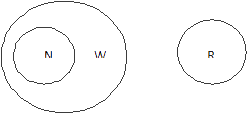# ZS-Associates Reasoning Question

DIRECTIONS for questions 1 to 4: What will come in place of question mark (?) in the given number series?
1. 15 27 37 45 51 ?
1. 158
2. 80
3. 65
4. 74
5. 55
Given series is: 15 27 37 45 51 ?
And the pattern is 27 – 15 = 12, 37 – 27 = 10, 45 – 37 = 8, 51 – 45 = 6.
2. 700 457 376 349 340 ?
1. 266
2. 329
3. 304
4. 337
5. 307
Given series is:
700 457 376 349 340 ?
Pattern is 700 – 457 = 243, 457 – 376 = 81, 376 – 349 = 27, 349 – 340 = 9
Thus, the next term is 340 – 3 = 337
1. 1 2 6 21 88 ?
1. 425
2. 475
3. 295
4. 445
5. 395
Given series is:
1 2 6 21 88 ?
Pattern is 1×1+1= 2
2×2+2= 6
6×3+3=21
21×4+4= 88
88×5+5= 445
So the next term of the series is = 445
2. 19 20 16 25 9 ?
1. 45
2. 55
3. 59
4. 34
5. 81
Given series is:
19 20 16 25 9 ?
Pattern is 19 – 20 = -1
20 – 16 = 4
16 – 25 = -9
25 – 9 = 16
So, next term of the series is = 9 – (-25) = 34
DIRECTIONS for question 5 to 7: The questions below has two/three statements followed by two conclusions numbered I and II.You have to take the given statements to be true even if they seem to be at variance with commonly known facts. Read all the conclusions and then decide which of the given conclusions logically follow from the given statements disregarding commonly known facts.
1. If only (1) conclusion follows
2. If only () conclusion follows
3. If either (1) or (2) follows
4. If neither (1) nor (2) follows
5. If both (1) and (2) follow
3. Statements: Some costs are tariffs. Some tariffs are fees.
1. Conclusion I: Some costs are fees.
2. Conclusion II: All costs are fees.Conclusion I: Some costs are fees, is false.
Conclusion II: All costs are fees, is false.
Neither conclusion I nor Conclusion II follows
4. Statements: All notices are warnings. No warning is a remark.
1. Conclusion I: Atleast some warnings are notices.
2. Conclusion II: No notice is a remark.Conclusion I: Atleast some warnings are notices, is true.
Conclusion II: No notice is a remark, is true.
Both conclusion I and II follows.
5. Statements: No thought is a perception. Some perceptions are suggestions.
1. Conclusion I: No thought is a suggestion.
2. Conclusion II: Some thoughts are suggestions.Conclusion I: No thought is a suggestion.
Conclusion II: Some thoughts are suggestions.
Individually conclusions I and II may or may not follow but either Conclusion I or II always follows.
DIRECTIONS for question 8 to 10: Study the following information and answer the question.
Nine friends- P, Q, R, S, T, U, V, W and X live on nine different floors of a building but not necessarily in the same order. The lower most floor of the building is numbered one, the one above that is numbered two and so on till the topmost floor is numbered nine. Only two persons live below the floor on which V lives. Only one person lives between V and P. W lives on an odd numbered floor but not on floor no. 7. Only two persons live between W and Q. X does not live on the topmost floor. P does not live on the lowermost floor. S lives immediately below R but R does not sit on topmost floor. Neither R nor T live on floor no. 6. U lives immediately above P
6. How many persons live between the floors on which P and S live?
1. Three
2. More than three
3. None
4. Two
5. One
The following arrangement can be made with the help of given information:
Floor No. Name
9 T
8 R
7 S
6 U
5 P
4 Q
3 V
2 X
1 W

Only one person lives between the floors on which P and S live.
7. Who lives on the floor immediately below V?
1. U
2. T
3. S
4. Q
5. X
The following arrangement can be made with the help of given information:
Floor No. Name
9 T
8 R
7 S
6 U
5 P
4 Q
3 V
2 X
1 W

X lives on the floor immediately below V.
8. On which of the following floor numbers does X live?
1. Four
2. One
3. Two
4. Five
5. Seven